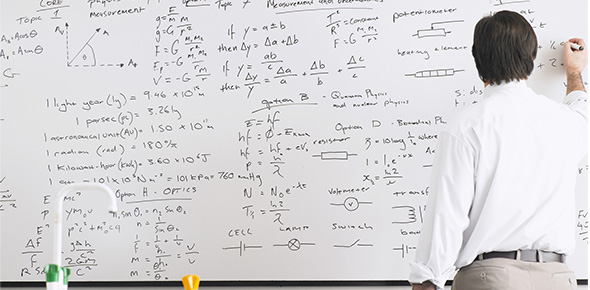# Balancing Equation And Conservation Of Matter

8 Questions | Attempts: 847
ShareSettingsThis quiz is designed to help you master the concepts of conservation of matter and become more comfortable with balancing equations.

• 1.
What does the Law of Conservation of Matter refer to?
• A.

Matter can not be created or destroyed.

• B.

Matter can be created but can not be destroyed.

• C.

Matter can be destroyed but not created.

• 2.
What is Matter?
• 3.
What side of the equation are the reactants on?
• A.

Right

• B.

Left

• C.

Middle

• D.

Both.

• 4.
What are the reactants in the following equation?H2 + O → H2
• A.

Hydrogen

• B.

Oxygen

• C.

Water

• D.

Hydrogen and Oxygen

• 5.
How many atoms of N are in the following molecule?NH3
• A.

1

• B.

2

• C.

3

• D.

0

• 6.
How many atoms of Fe are in the following molecule?Fe2O3
• A.

0

• B.

1

• C.

2

• D.

3

• 7.
Is the following equation balanced? NH3 + HCl à   NH4Cl          (yes or no)
• 8.
What are the correct coefficients needed to balance the following equation?____Al + _____ O2 à ____ Al2O3
• A.

2,3,4

• B.

1,3,2

• C.

4,3,2

• D.

4,2,3

## Related TopicsBack to top
×

Wait!
Here's an interesting quiz for you.